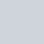# Independent Component Analysis (ICA) using R

ICA means Independent Component Analysis. ICA is the most powerful and widely used statistical technique which is used to separate independent sources from their mixture. It is also known as blind source separation technique.## The Situation where we use ICA

We can easily simplify the situation where we use ICA by the following example,

Imagine two people having a conversation at a cocktail party. For whatever reason, we have two microphones placed near both party-goers. Both voices are heard by both microphones at different volumes based on the distance between the person and microphone. In other words, we record two files that include audio from the two party-goers mixed together. In this situation, we can easily separate their voices using ICA. But we have to ensure that the mixed signal have following properties:

• Independence: if the source signals are independent, their mixture signals are not. This is because the source signals are shared between both mixtures.
• Gaussianity/Normality: The histogram of mixed signals is bell-shaped histogram, Gaussian or normal. The source signals must be non-Gaussian, and this assumption is a fundamental restriction in ICA. Hence, the ICA model cannot estimate Gaussian independent components.
• Complexity: Mixed signal must be more complex than source signal.

## Performing Independent Component Analysis (ICA) using R

We can easily perform ICA using R or R studio. To perform ICA, we can use fastICA R package. We have to install fastICA package in R or R studio.

fastICA package

fastICA(X, n.comp, alg.typ = c(“parallel”,”deflation”),
fun = c(“logcosh”,”exp”), alpha = 1.0, method = c(“R”,”C”),
row.norm = FALSE, maxit = 200, tol = 1e-04, verbose = FALSE,
w.init = NULL)

The Arguments of the fastICA package are as follows,

 Arguments Meaning of the Arguments X A data matrix with n rows representing observations and p columns representing variables. n.comp Number of components to be extracted. Alg.typ If alg.typ == “parallel” the components are extracted simultaneously (the default). if alg.typ == “deflation” the components are extracted one at a time. fun The functional form of the G function used in the approximation to neg-entropy. alpha Constant in range [1, 2] used in approximation to neg-entropy when fun == “logcosh”. method If method == “R” then computations are done exclusively in R (default). The code allows the interested R user to see exactly what the algorithm does. If method == “C” then C code is used to perform most of the computations, which makes the algorithm run faster. During compilation the C code is linked to an optimized BLAS library if present, otherwise stand-alone BLAS routines are compiled. row.norm A logical value indicating whether rows of the data matrix X should be standardized beforehand. maxit Maximum number of iterations to perform ICA. tol A positive scalar giving the tolerance at which the un-mixing matrix is considered to have converged. verbose A logical value indicating the level of output as the algorithm runs. w.init Initial un-mixing matrix of dimension c(n.comp, n.comp). If NULL (default) then a matrix of normal r.v.’s is used.

## Steps Involved in ICA

The following steps are maintained when one performed ICA in R.

1. Centering
2. Whitening
3. Symmetric FastICA using logcosh approx. to neg-entropy function
4. Iteration 1 tol = 0.1186187
5. Iteration 2 tol = 0.002116623
6. Iteration 3 tol = 2.003116e-06

### R Code-1: un-mixing two mixed independent uniforms using R

``````#R Code 1: un-mixing two mixed independent uniforms

S <- matrix(runif(10000), 5000, 2)
A <- matrix(c(1, 1, -1, 3), 2, 2, byrow = TRUE)
X <- S %*% A
a <- fastICA(X, 2, alg.typ = "parallel", fun = "logcosh", alpha = 1,
method = "C", row.norm = FALSE, maxit = 200,
tol = 0.0001, verbose = TRUE)
par(mfrow = c(1, 3))
plot(a\$X, main = "Pre-processed data")
plot(a\$X %*% a\$K, main = "PCA components")
plot(a\$S, main = "ICA components")
``````

### R Code-2: un-mixing two independent signals using R

``````#R Code 2: un-mixing two independent signals

S <- cbind(sin((1:1000)/20), rep((((1:200)-100)/100), 5))
A <- matrix(c(0.291, 0.6557, -0.5439, 0.5572), 2, 2)
X <- S %*% A
a <- fastICA(X, 2, alg.typ = "parallel", fun = "logcosh", alpha = 1,
method = "R", row.norm = FALSE, maxit = 200,
tol = 0.0001, verbose = TRUE)
par(mfcol = c(2, 3))
plot(1:1000, S[,1 ], type = "l", main = "Original Signals",
xlab = "", ylab = "")
plot(1:1000, S[,2 ], type = "l", xlab = "", ylab = "")
plot(1:1000, X[,1 ], type = "l", main = "Mixed Signals",
xlab = "", ylab = "")
plot(1:1000, X[,2 ], type = "l", xlab = "", ylab = "")
plot(1:1000, a\$S[,1 ], type = "l", main = "ICA source estimates",
xlab = "", ylab = "")
plot(1:1000, a\$S[, 2], type = "l", xlab = "", ylab = "")
``````

### R Code-3: Using FastICA to perform projection pursuit on a mixture of bivariate normal distributions using R

``````#R Code 3: using FastICA to perform projection pursuit on a mixture of bivariate normal distributions

if(require(MASS)){
x <- mvrnorm(n = 1000, mu = c(0, 0), Sigma = matrix(c(10, 3, 3, 1), 2, 2))
x1 <- mvrnorm(n = 1000, mu = c(-1, 2), Sigma = matrix(c(10, 3, 3, 1), 2, 2))
X <- rbind(x, x1)
a <- fastICA(X, 2, alg.typ = "deflation", fun = "logcosh", alpha = 1,
method = "R", row.norm = FALSE, maxit = 200,
tol = 0.0001, verbose = TRUE)
par(mfrow = c(1, 3))
plot(a\$X, main = "Pre-processed data")
plot(a\$X %*% a\$K, main = "PCA components")
plot(a\$S, main = "ICA components")
}
``````

#### Result of ICAThe above code containing the following components,

 Components X Pre-processed data matrix. K Pre-whitening matrix that projects data onto the first n.comp principal components. W Estimated un-mixing matrix. A Estimated mixing matrix. S Estimated source matrix.

You can also find some other data analysis content here.

Data analysis using SPSS

SPSS tutorials

Canonical Correlation Analysis (CCA)

Principle Component Analysis (PCA)

Independent component analysis (ICA)

### 2 thoughts on “Independent Component Analysis (ICA) using R”

1.Vapor Store

2.먹튀검증 먹튀폴리스